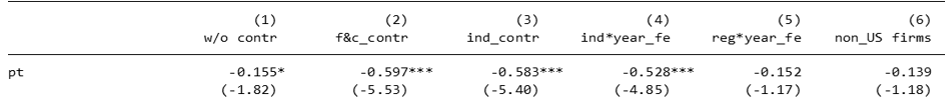# #StackBounty: #econometrics #subsampling What we should do when the result is only significant to one country but the whole sample?

### Bounty: 100

In a staggered Difference-in-Differences setting, Dasgupta, 2019 has a formula for such a static setting is

$$Y_{it}$$ = $$alpha$$ + $$beta$$ $$pt_{kt}$$ + $$deltaX_{ikt}$$ + $$theta_t$$ + $$gamma_i$$ +$$epsilon_{it}$$ (1)

where $$i$$, $$k$$, and $$t$$ index firms, countries, and years respectively. $$X_{ikt}$$ is a vector of the different firm, country, and industry control, while $$gamma$$ and $$theta$$ are firm and year fixed effects.

When replicating table 3 in his paper, I focus on columns (2) and (6). In these two columns, I have the same control variables sets, fixed effects sets, but only difference in sample. While columns (2) controlled for the whole sample but column (6) controlled for non-US sample.As we can see from the Table, the coefficients of $$pt_{kt}$$ tell us the effect of laws stronger in US countries but weaker in non-US countries. So, I need to address this issue

What I tried is that I add US_dummy equalling to 1 to specification (2) if this firm is in the US to the equation. But I faced the omitted issue because the the information in US_dummy is carried by the year fixed effect already.

That said, I simply cannot solve the weakened results in non-US sample problem by using the US_dummy in all regressions.
Can you suggest me any other way to deal with this issue?

Get this bounty!!!

This site uses Akismet to reduce spam. Learn how your comment data is processed.